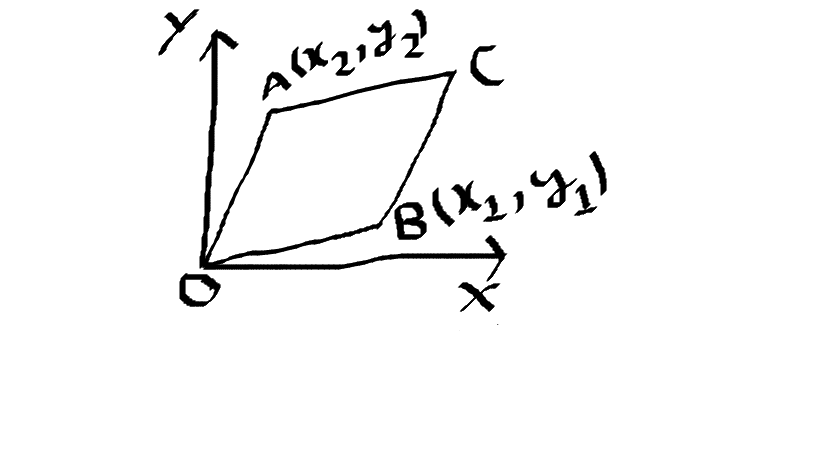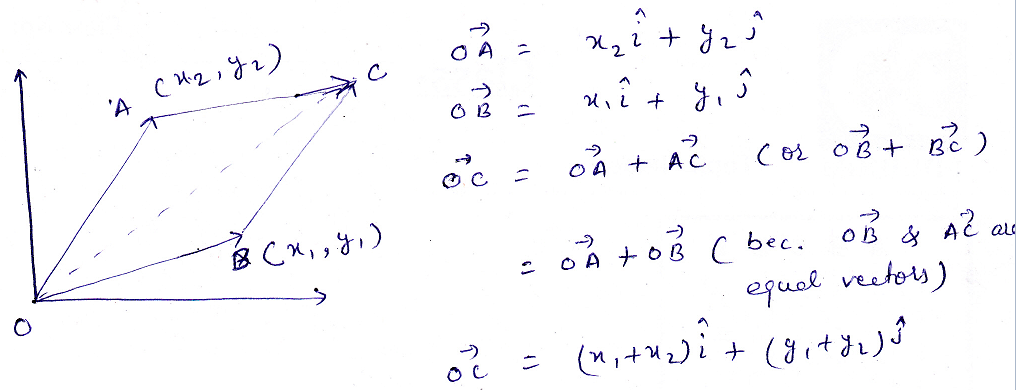# Vector problem

Using vectors prove that :the co-ordinates of C is (x1+x2,y1+y2)

Given : the coordinates of A are (x2,y2) and the coordinates of B are (x1,y1) and OACB is a parallelogram

(Y and X represent the coordinate axes)

•